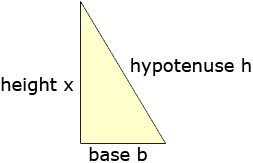Name: kelsey Who is asking: Student Level of the question: All Question: how can i find the height of a triangle if i have the base and the hypotenuse Hi Kelsey, You used the word hypotenuse so I am assuming that you have a right triangle. If so you can use Pythagoras Theorem.If you have a right triangle with base of length b units, height of x units and a hypotenuse of h units then Pythagoras Theorem states that b2 + x2 = h2 Substitute the values you have for b and h and solve for x. If the triangle is not a right triangle then you need more information. If you only know the lengths of two sides then you can construct many different triangles with those side lengths. There is more on an explanationin the answer to a previous question. Penny